Ex 13.3

Chapter 13 Class 10 Surface Areas and Volumes
Serial order wise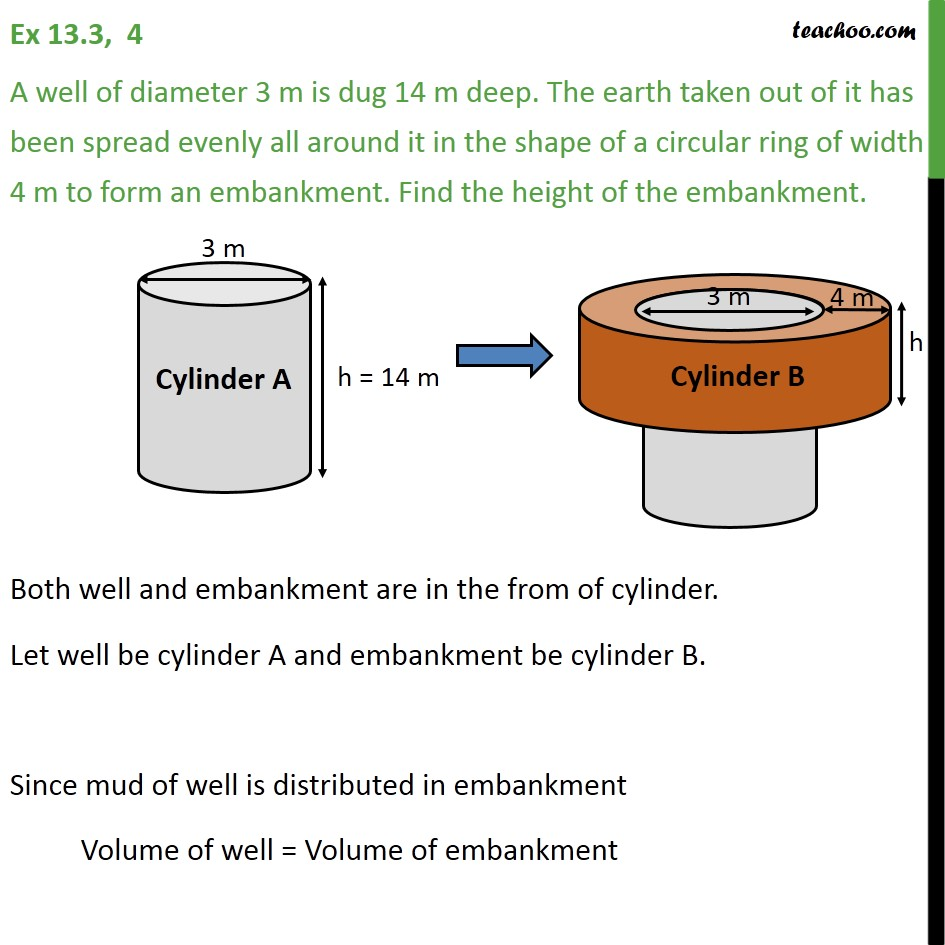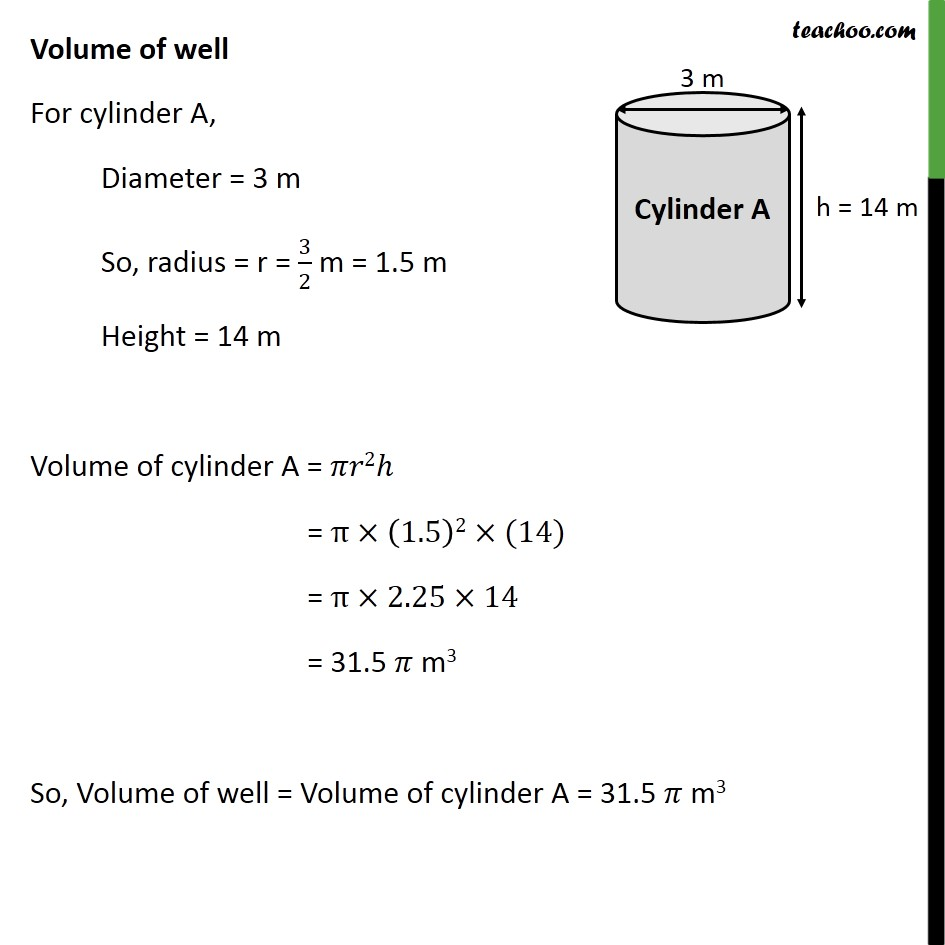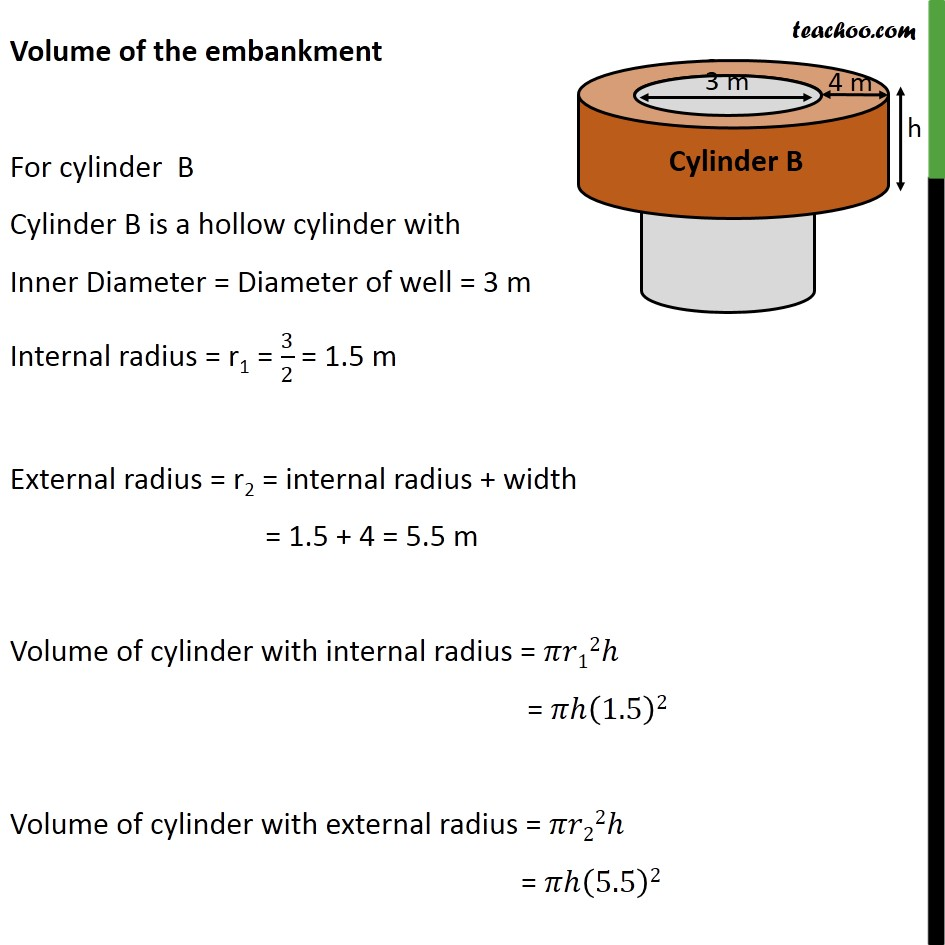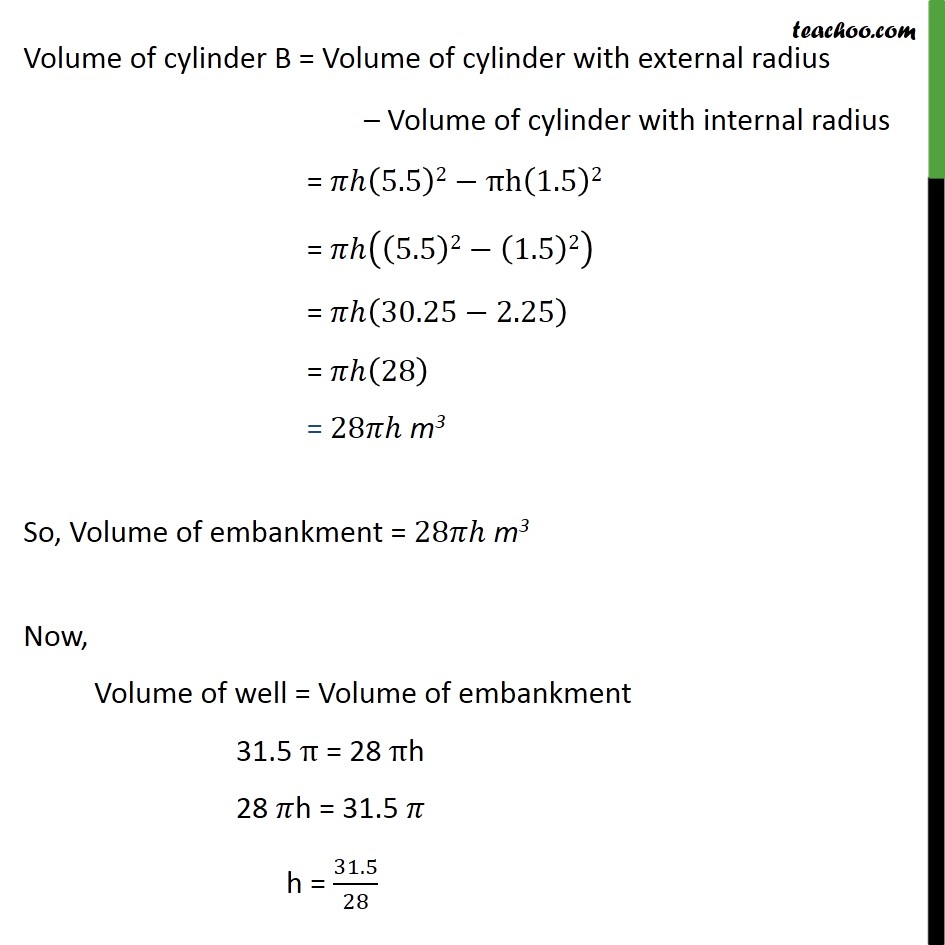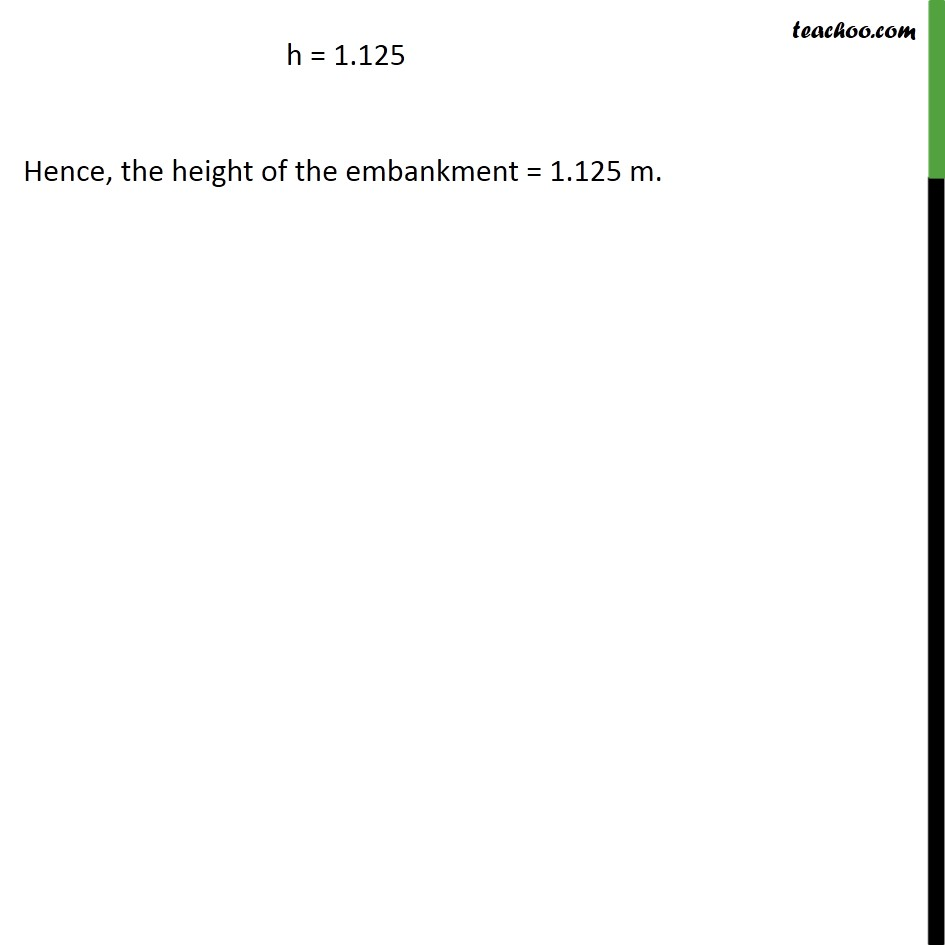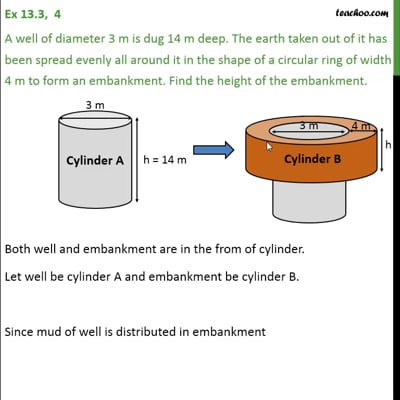This video is only available for Teachoo black users

Maths Crash Course - Live lectures + all videos + Real time Doubt solving!

### Transcript

Ex 13.3, 4 A well of diameter 3 m is dug 14 m deep. The earth taken out of it has been spread evenly all around it in the shape of a circular ring of width 4 m to form an embankment. Find the height of the embankment. Both well and embankment are in the from of cylinder. Let well be cylinder A and embankment be cylinder B. Since mud of well is distributed in embankment Volume of well = Volume of embankment Volume of well For cylinder A, Diameter = 3 m So, radius = r = 3/2 m = 1.5 m Height = 14 m Volume of cylinder A = 2 = (1.5)2 (14) = 2.25 14 = 31.5 m3 So, Volume of well = Volume of cylinder A = 31.5 m3 Volume of the embankment For cylinder B Cylinder B is a hollow cylinder with Inner Diameter = Diameter of well = 3 m Internal radius = r1 = 3/2 = 1.5 m External radius = r2 = internal radius + width = 1.5 + 4 = 5.5 m Volume of cylinder with internal radius = 12 = (1.5)2 Volume of cylinder with external radius = 22 = (5.5)2 Volume of cylinder B = Volume of cylinder with external radius Volume of cylinder with internal radius = (5.5)2 h(1.5)2 = ((5.5)2 (1.5)2) = (30.25 2.25) = (28) = 28 m3 So, Volume of embankment = 28 m3 Now, Volume of well = Volume of embankment 31.5 = 28 h 28 h = 31.5 h = 31.5/28 h = 1.125 Hence, the height of the embankment = 1.125 m.